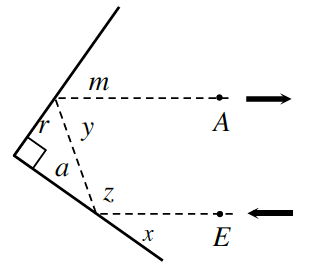### Home > CCG > Chapter 7 > Lesson 7.2.2 > Problem7-67

7-67.

A light directed from point $E$ is pointed at a hinged mirror with right angle as shown at right.

1. If $∠x$ measures $36°$, find the measures of $∠a$, $∠r$, $∠m$, $∠y$, and $∠z$.

When the light hits the mirror the angle of incidence and angle of reflection are congruent. Therefore $∠x=∠a$

$∠a=36°$, $∠r=54°$, $∠m=54°$, $∠y=72°$$∠z=108°$

2. Why must the arrows at points $A$ and $E$ be parallel?

Use the angle measurements found to explain why the arrows at points $A$ and $E$ must be parallel.